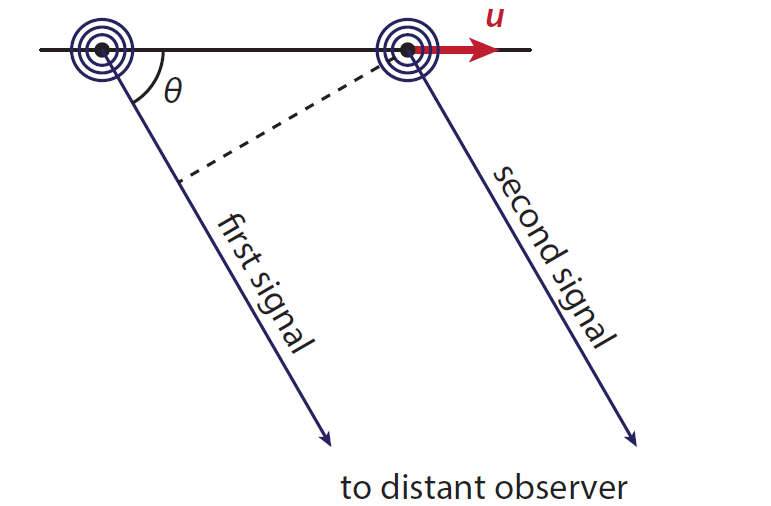# 15.E: Relativistic Forces and Waves (Exercises)

$$\newcommand{\vecs}{\overset { \rightharpoonup} {\mathbf{#1}} }$$ $$\newcommand{\vecd}{\overset{-\!-\!\rightharpoonup}{\vphantom{a}\smash {#1}}}$$$$\newcommand{\id}{\mathrm{id}}$$ $$\newcommand{\Span}{\mathrm{span}}$$ $$\newcommand{\kernel}{\mathrm{null}\,}$$ $$\newcommand{\range}{\mathrm{range}\,}$$ $$\newcommand{\RealPart}{\mathrm{Re}}$$ $$\newcommand{\ImaginaryPart}{\mathrm{Im}}$$ $$\newcommand{\Argument}{\mathrm{Arg}}$$ $$\newcommand{\norm}{\| #1 \|}$$ $$\newcommand{\inner}{\langle #1, #2 \rangle}$$ $$\newcommand{\Span}{\mathrm{span}}$$ $$\newcommand{\id}{\mathrm{id}}$$ $$\newcommand{\Span}{\mathrm{span}}$$ $$\newcommand{\kernel}{\mathrm{null}\,}$$ $$\newcommand{\range}{\mathrm{range}\,}$$ $$\newcommand{\RealPart}{\mathrm{Re}}$$ $$\newcommand{\ImaginaryPart}{\mathrm{Im}}$$ $$\newcommand{\Argument}{\mathrm{Arg}}$$ $$\newcommand{\norm}{\| #1 \|}$$ $$\newcommand{\inner}{\langle #1, #2 \rangle}$$ $$\newcommand{\Span}{\mathrm{span}}$$$$\newcommand{\AA}{\unicode[.8,0]{x212B}}$$

15.1 Two spaceships are connected by a strong cable. Both ships are initially at rest, and ignite their engines at the same time. Their accelerations are identical at every point in time. Does the cable stay intact? Hint: use a spacetime diagram to analyze what happens.

15.2 In classical mechanics, the Doppler shift in the wavelength of an object moving towards the observer at
speed u is given by equation (9.7.1) repeated again below:

$\lambda_{\mathrm{obs}}=\frac{v-u}{v} \lambda\ \label{15.E.1}$

where $$v$$ is the speed of the wave (usually sound). To get this result, we compared what happens with the source and the wave in a fixed time interval $$\Delta t$$. As you now know, this result cannot hold at speeds close to that of light, because in that case there will be a significant effect due to time dilation. In this problem, we’ll, therefore, redo the calculation to account for relativistic effects.

We consider a distant source of light that moves with velocity $$\boldsymbol{u}$$. At time $$t = 0$$ (for both the source and the stationary observer), the source emits a signal (this could be a wave crest, but the argument holds for any signal). A time $$\Delta t^{\prime}$$) later, as measured on the clock moving with the source, the source emits a second signal, see Figure 15.E.1.Figure $$PageIndex{1}$$: Setting for calculating the relativistic Doppler effect. As the (stationary) observer is distant, the signals traveling to the observer from the source can be taken parallel to each other; they make an angle $$\theta$$ with the direction in which the source moves. Note that both the distance between the position of the source at the emission of the first and second signal and the difference in distance traveled by the signal matter.
1. Determine the time interval $$\Delta t$$ between the emission of the first and second signal as measured on the clock of the stationary observer.
2. Determine the change in distance $$\Delta x$$ between the (stationary) observer and the (moving) source in the time interval between the two signals, as measured by the stationary observer.
3. Now determine the time interval $$\Delta t_{obs}$$ between the arrival of the first and second signal at the location of the observer.
4. From your answers at (a-c), show that the observed frequency $$\nu_{obs}$$ is related to the source’s frequency $$\nu_{s}$$ through $v_{\mathrm{obs}}=\frac{\sqrt{1-u^{2} / c^{2}}}{1+(u / c) \cos \theta} v_{\mathrm{s}}\label{15.E.2}$ Note that equation (\ref{15.E.2}) reduces to (\ref{15.E.1}) in the case that the source is moving radially away from the observer.
5. From equation (\ref{15.E.2}), find the expression for the (relativistic) transverse Doppler shift for the case that the source is moving in a direction perpendicular to the observer’s line of sight (i.e., $$\theta = 90^{o}$$). How can you tell that in this case, the Doppler shift is exclusively due to time dilation?

This page titled 15.E: Relativistic Forces and Waves (Exercises) is shared under a CC BY-NC-SA 4.0 license and was authored, remixed, and/or curated by Timon Idema (TU Delft Open) via source content that was edited to the style and standards of the LibreTexts platform; a detailed edit history is available upon request.